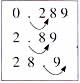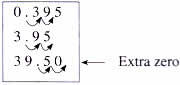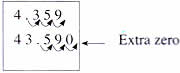Thursday , July 7 2022# NCERT 5th Class (CBSE) Mathematics: Decimals

### Multiplication by 10,100,1000

Look at these examples.

10×7.623 = 76.23

100×7.623 = 762.3

1000×7.623 = 7623

#### Multiplying by 10 moves the decimal point one place to the right.

100.289 = 02.89

10×2.89 = 28.9

10×28.9 = 289#### Multiplying by 100 moves the decimal point two places to the right.

100×0.395 = 39.5

100×3.95 = 395

100×39.5 = 3950#### Multiplying by 1000 moves the decimal point three places to the right.

1000×4.359 = 4359

1000×43.59 = 43590## चुनौती हिमालय की 5th NCERT CBSE Hindi Rimjhim Ch 18

चुनौती हिमालय की 5th Class NCERT CBSE Hindi Book Rimjhim Chapter 18 प्रश्न: लद्दाख जम्मू-कश्मीर राज्य में …